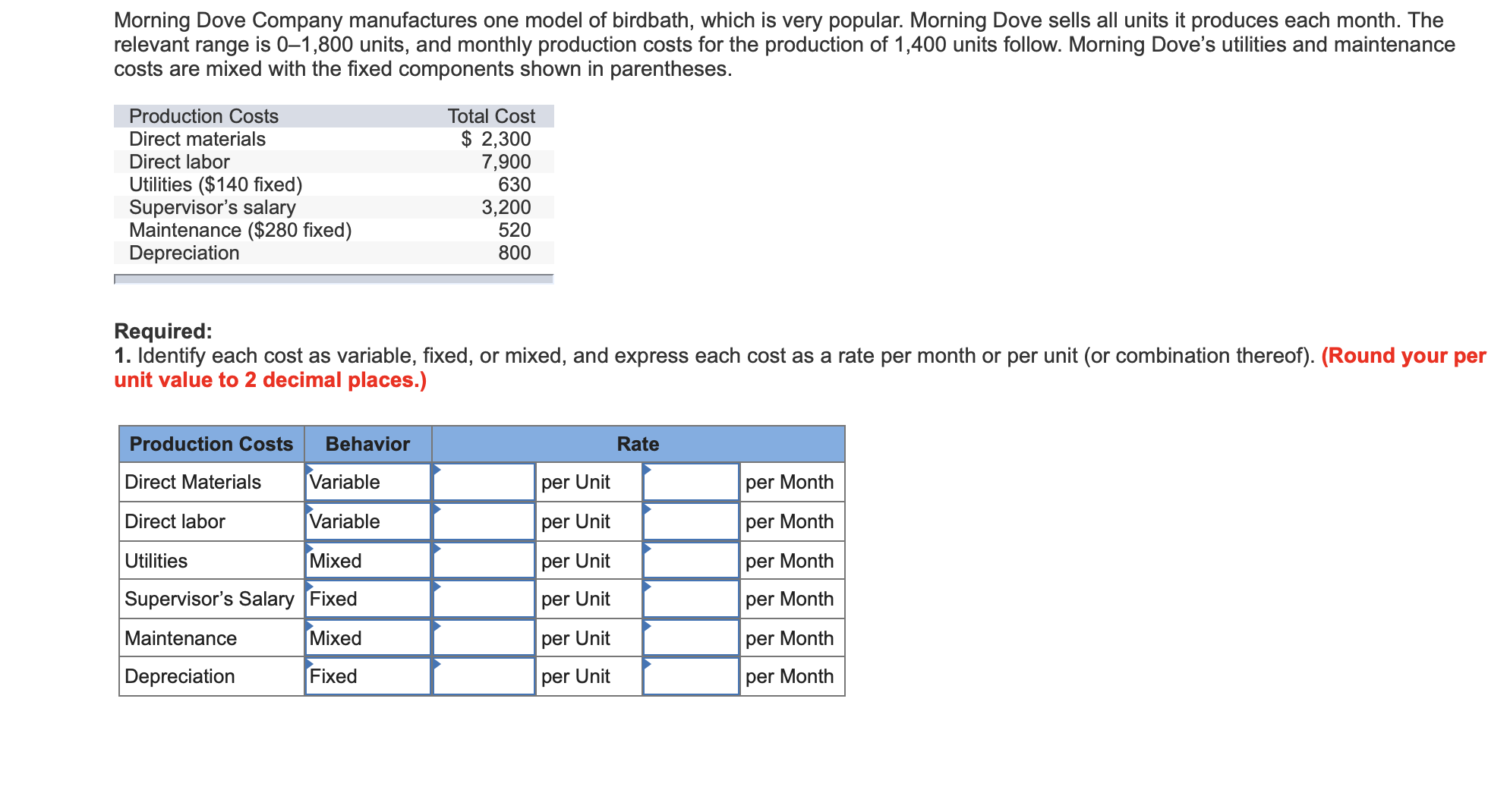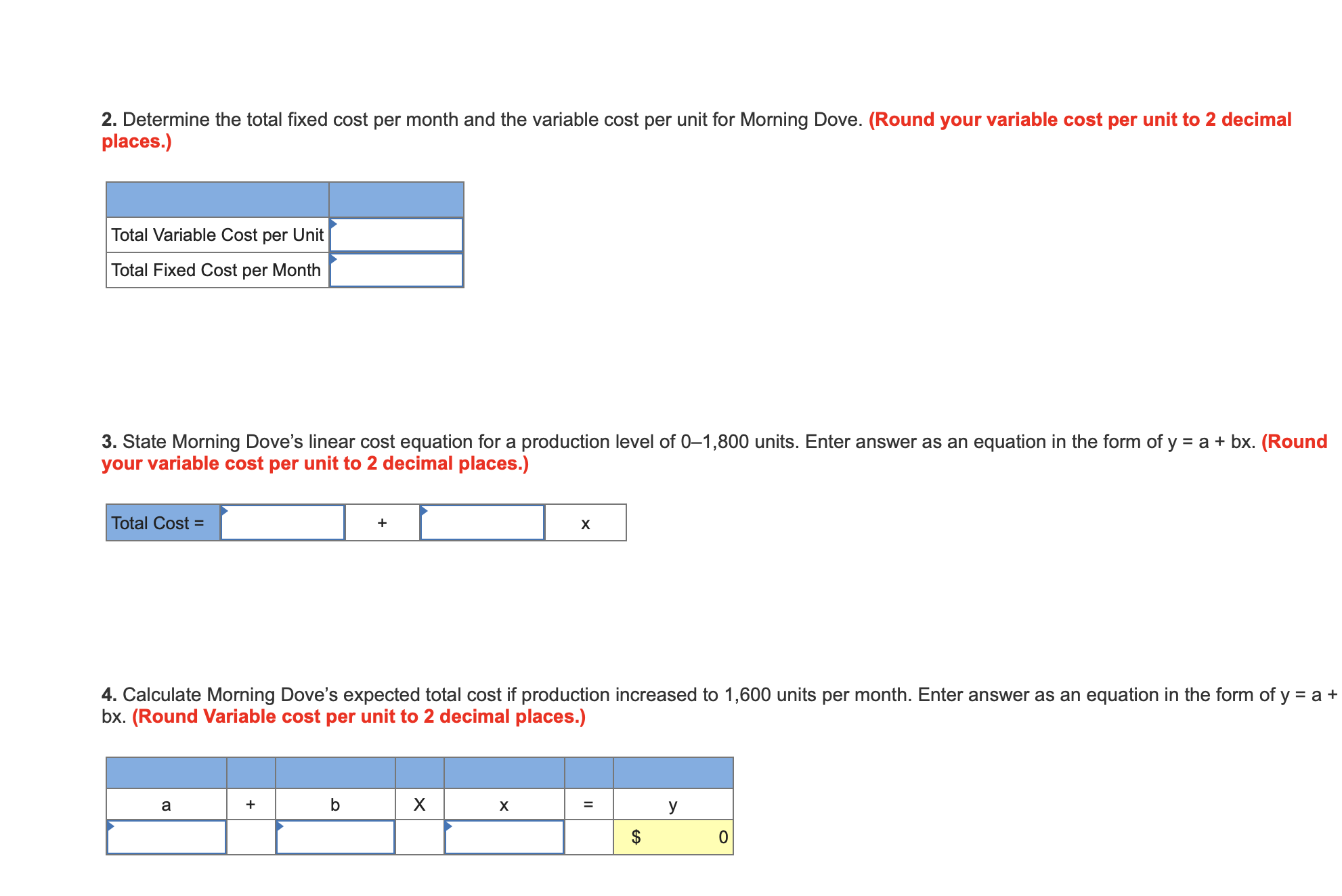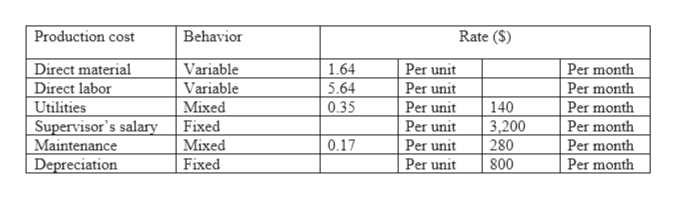Morning Dove Company manufactures one model of birdbath, which is very popular. Morning Dove sells all units it produces each month. Therelevant range is 0-1,800 units, and monthly production costs for the production of 1,400 units follow. Morning Dove's utilities and maintenancecosts are mixed with the fixed components shown in parentheses.Production CostsTotal Cost\$2,3007,9006303,200520800Direct materialsDirect laborUtilities (\$140 fixed)Supervisor's salaryMaintenance (\$280 fixed)DepreciationRequired:1. Identify each cost as variable, fixed, or mixed, and express each cost as a rate per month or per unit (or combination thereof). (Round your perunit value to 2 decimal places.)BehaviorProduction CostsRateDirect MaterialsVariableper Unitper MonthVariableDirect laborper Monthper UnitMixedper MonthUtilitiesper UnitSupervisor's Salary Fixedper Monthper UnitMixedMaintenanceper Monthper UnitFixedDepreciationper Unitper Month 2. Determine the total fixed cost per month and the variable cost per unit for Morning Dove. (Round your variable cost per unit to 2 decimalplaces.)Total Variable Cost per UnitTotal Fixed Cost per Month3. State Morning Dove's linear cost equation for a production level of 0-1,800 units. Enter answer as an equation in the form of yyour variable cost per unit to 2 decimal places.)abx. (RoundTotal Cost =X4. Calculate Morning Dove's expected total cost if production increased to 1,600 units per month. Enter answer as an equation in the form of y = a +bx. (Round Variable cost per unit to 2 decimal places.)X+аXУOее

Question

I keep getting this question wrong and trying to see how to work ithelp_outlineImage TranscriptioncloseMorning Dove Company manufactures one model of birdbath, which is very popular. Morning Dove sells all units it produces each month. The relevant range is 0-1,800 units, and monthly production costs for the production of 1,400 units follow. Morning Dove's utilities and maintenance costs are mixed with the fixed components shown in parentheses. Production Costs Total Cost \$2,300 7,900 630 3,200 520 800 Direct materials Direct labor Utilities (\$140 fixed) Supervisor's salary Maintenance (\$280 fixed) Depreciation Required: 1. Identify each cost as variable, fixed, or mixed, and express each cost as a rate per month or per unit (or combination thereof). (Round your per unit value to 2 decimal places.) Behavior Production Costs Rate Direct Materials Variable per Unit per Month Variable Direct labor per Month per Unit Mixed per Month Utilities per Unit Supervisor's Salary Fixed per Month per Unit Mixed Maintenance per Month per Unit Fixed Depreciation per Unit per Month fullscreenhelp_outlineImage Transcriptionclose2. Determine the total fixed cost per month and the variable cost per unit for Morning Dove. (Round your variable cost per unit to 2 decimal places.) Total Variable Cost per Unit Total Fixed Cost per Month 3. State Morning Dove's linear cost equation for a production level of 0-1,800 units. Enter answer as an equation in the form of y your variable cost per unit to 2 decimal places.) abx. (Round Total Cost = X 4. Calculate Morning Dove's expected total cost if production increased to 1,600 units per month. Enter answer as an equation in the form of y = a + bx. (Round Variable cost per unit to 2 decimal places.) X + а X У O ее fullscreen
Step 1

Production costs are such cost of a company which the company must be incurred for the manufacturing of a service or a product. It includes expenses of labor, raw material, salary of supervisor, depreciation.

Step 2

1

Given:

Total production units are 1,400.

The behavior of each cost and rate per unit or per month of each cost is as given below:help_outlineImage TranscriptioncloseProduction cost Behavior Rate (S) Variable Variable Міxed Per month Per month Per month Direct material Direct labor Utilities Supervisor's salary Maintenance Depreciation 1.64 Per unit Per unit Per unit Per unit Per unit 5.64 0.35 140 Fixed Міxed 3,200 280 Per month 0.17 Per month Per month Fixed Per unit 800 fullscreen
Step 3

Working notes:

• Direct material per unit rate: \$2,300/1,400 = \$1.64
• Direct labor per unit rate: \$7,900/1,400 = \$5.64
• Utilities: total amount is \$630. Fixed among this is \$140. Variable utilities are \$630-\$140 = \$490
• Variable utilities rate per unit: \$490/1,400 = 0.35
• Maintenan...

Want to see the full answer?

See Solution

Want to see this answer and more?

Our solutions are written by experts, many with advanced degrees, and available 24/7

See Solution
Tagged in